• f(t)为X的概率密度 注： 例题： 常见连续型随机变量的分布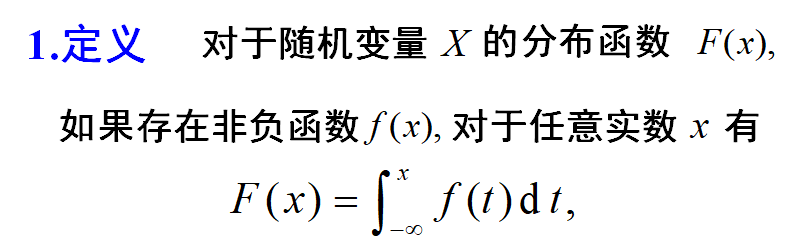f(t)为X的概率密度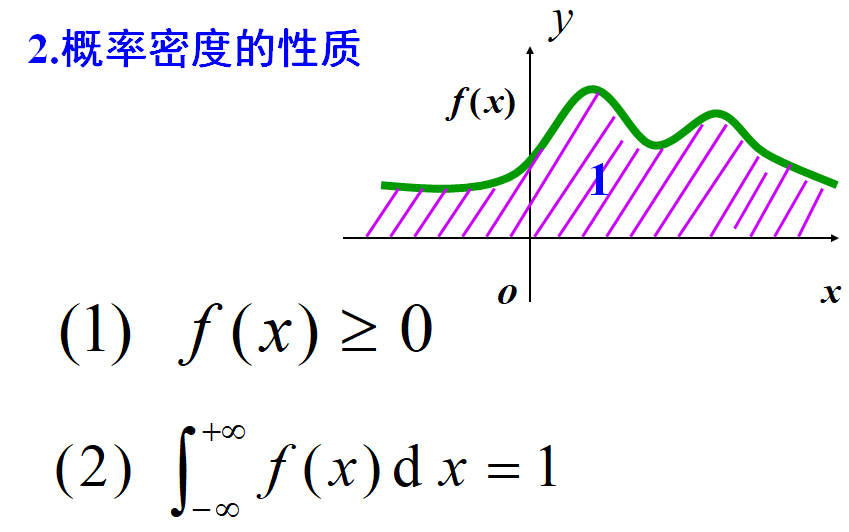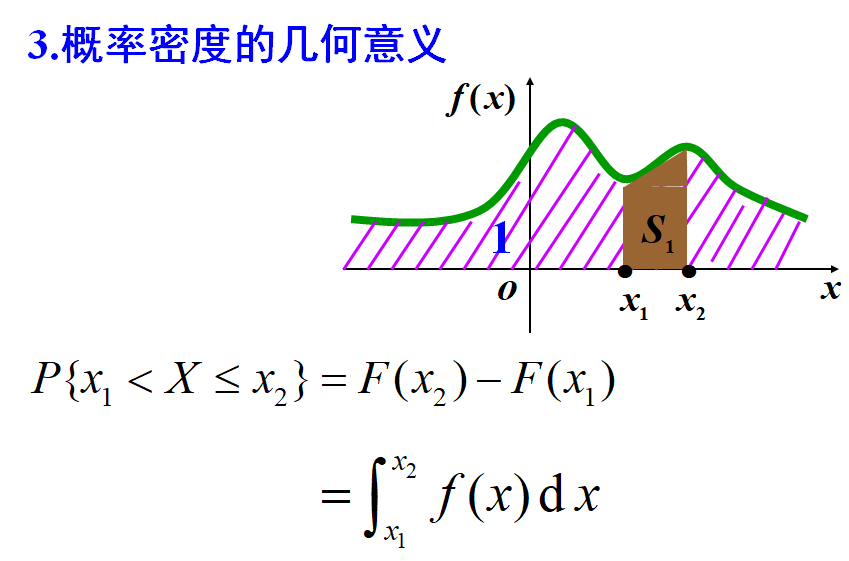注：例题：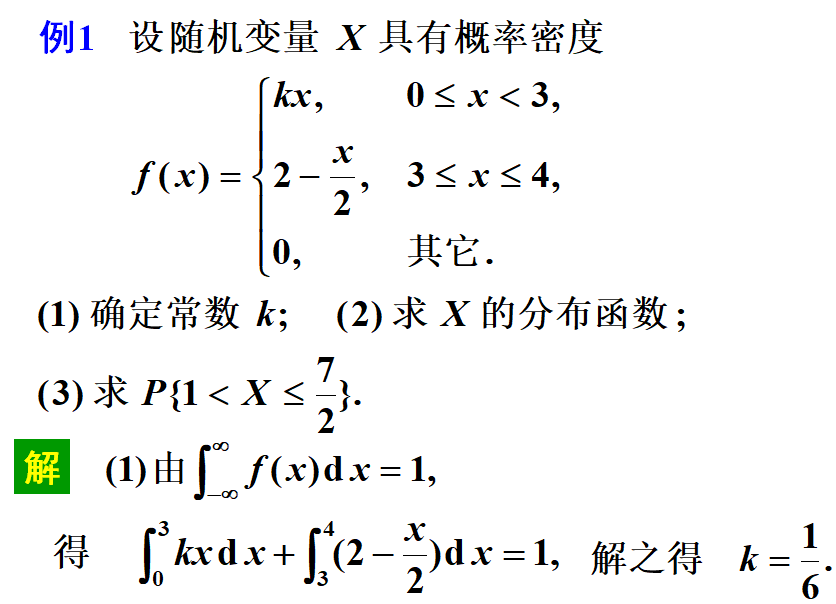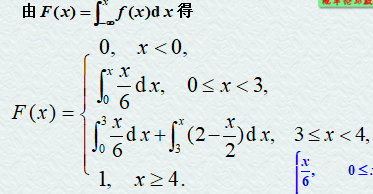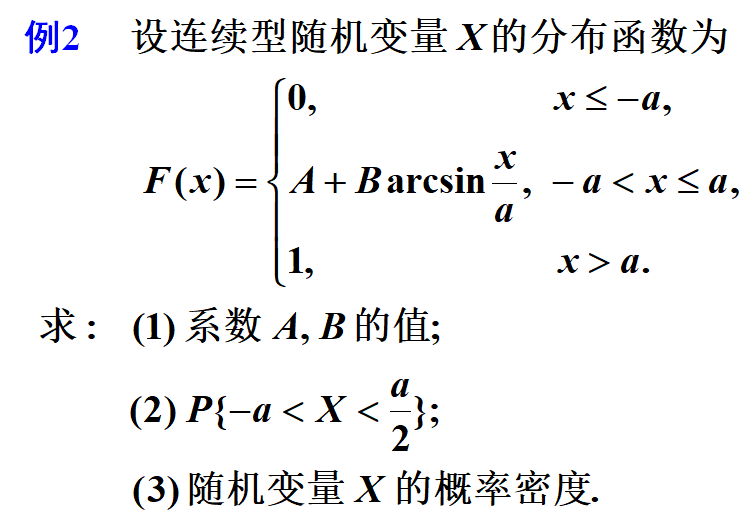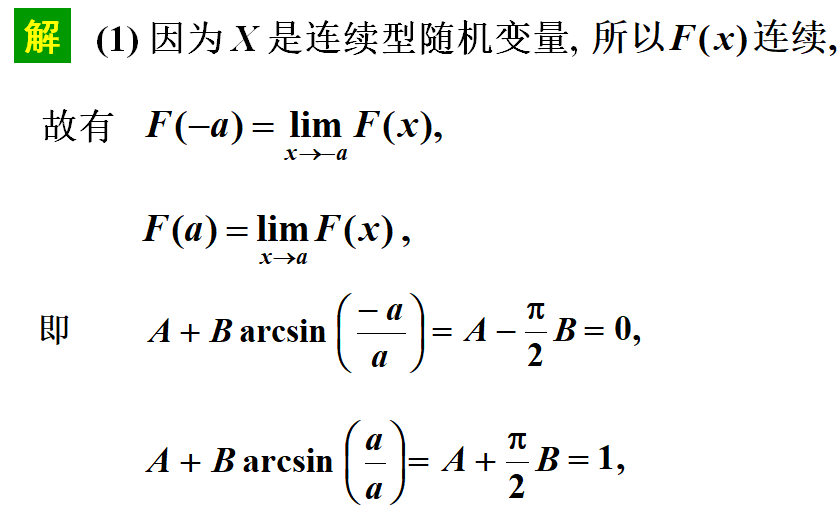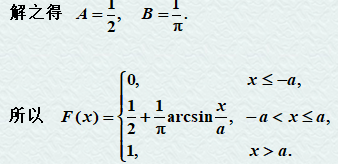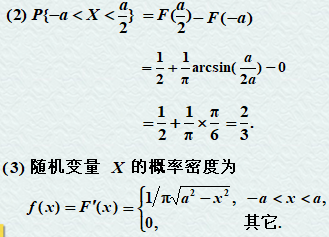常见连续型随机变量的分布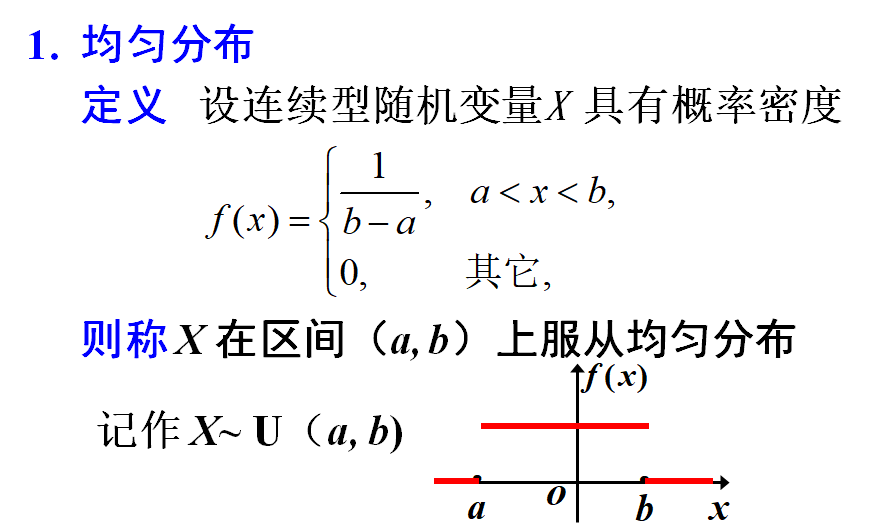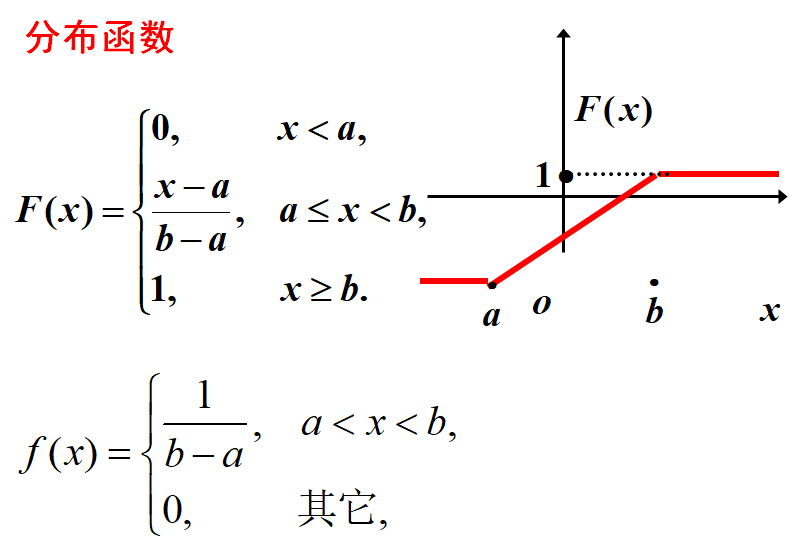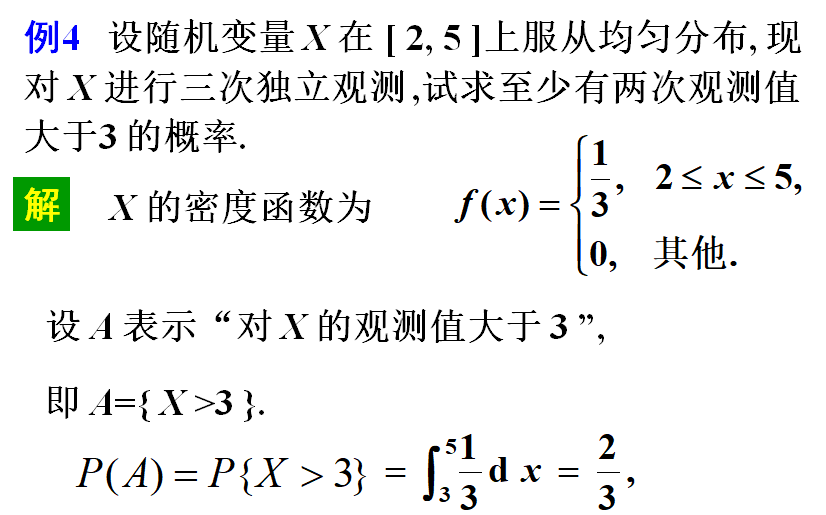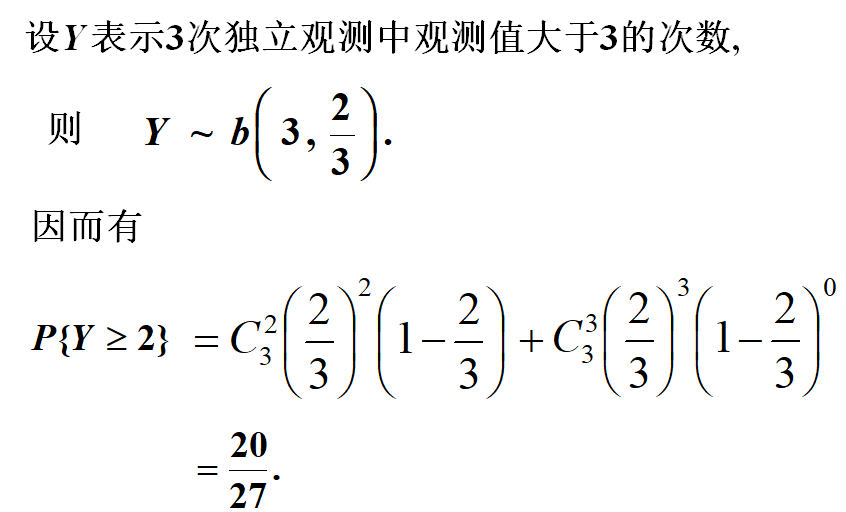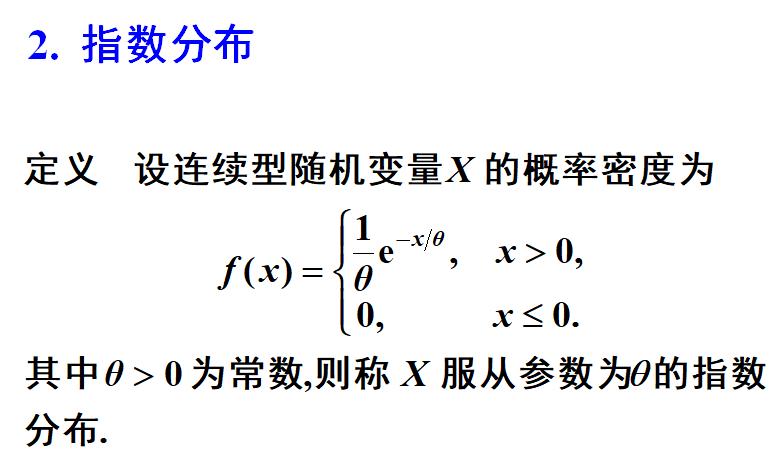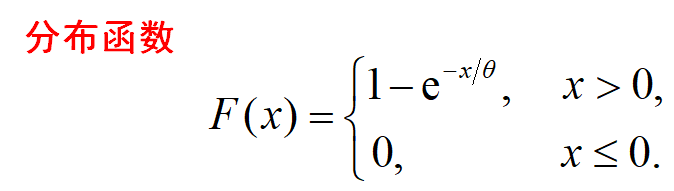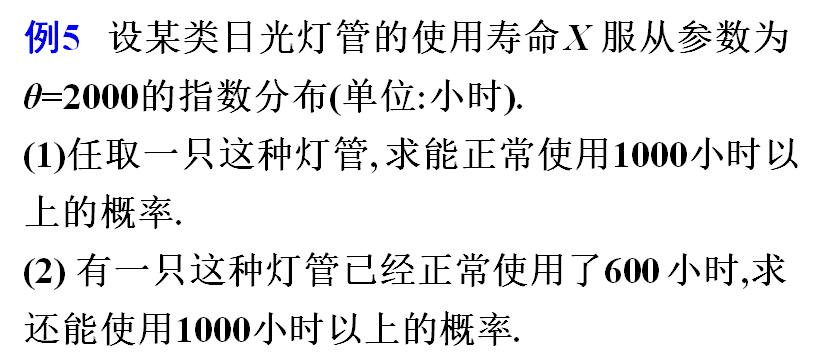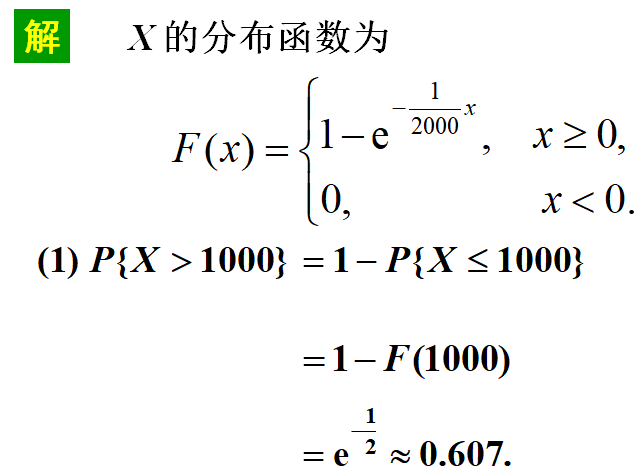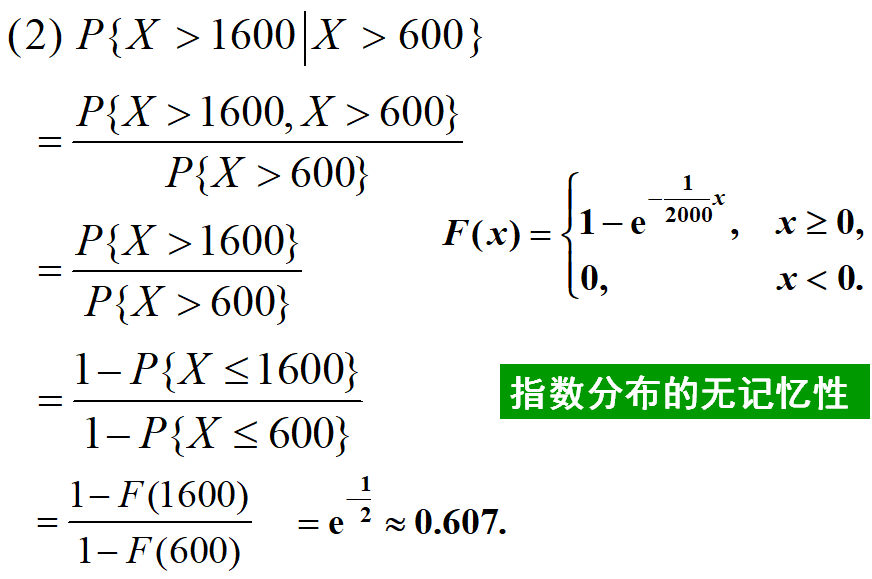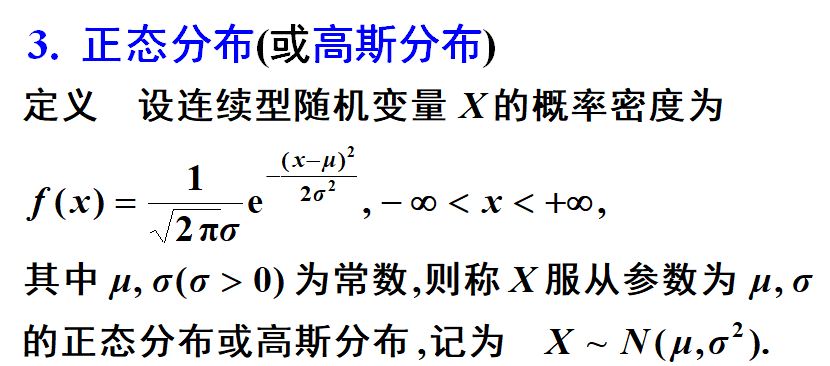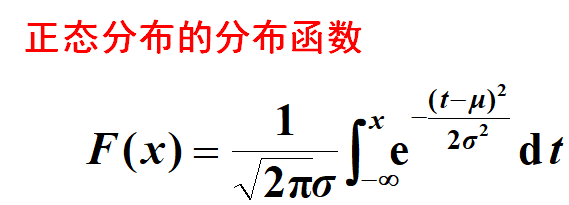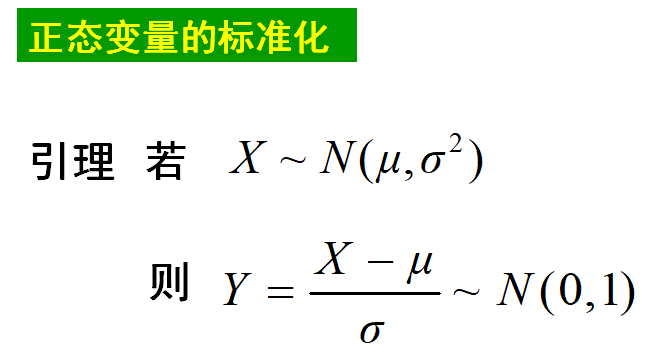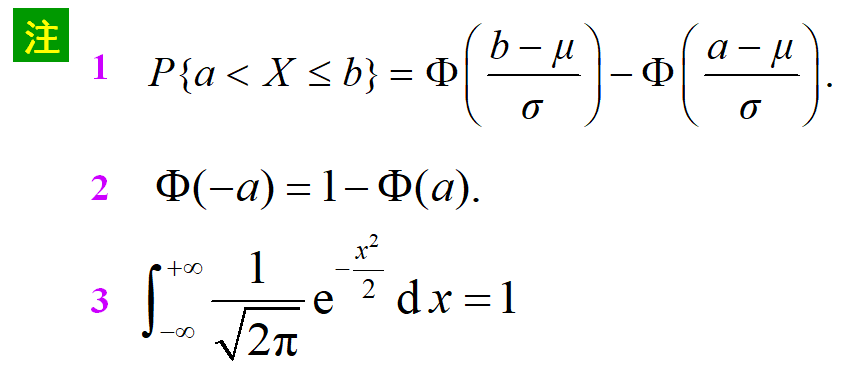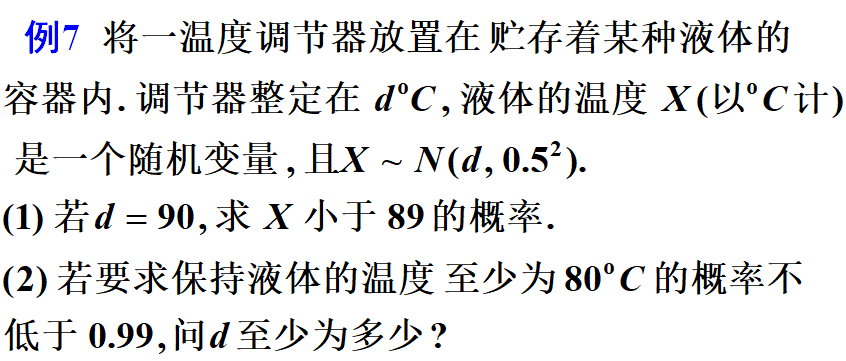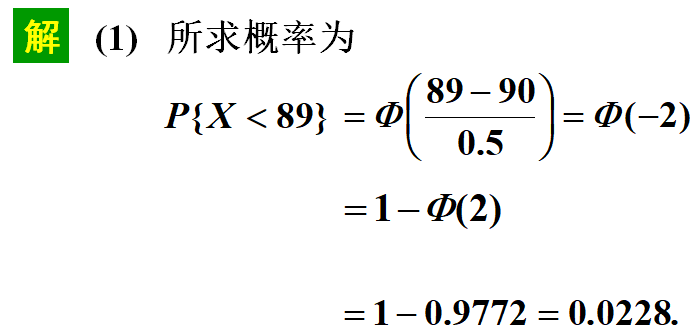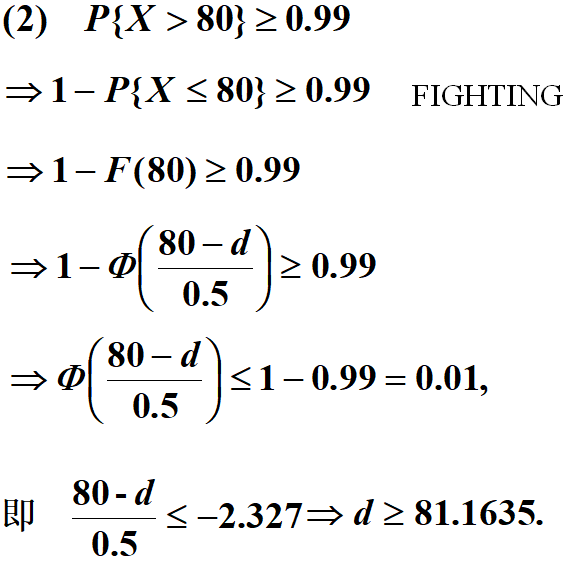展开全文• 概率密度的概念与性质 1.定义 2.性质 典型例题
概率密度的概念与性质 1.定义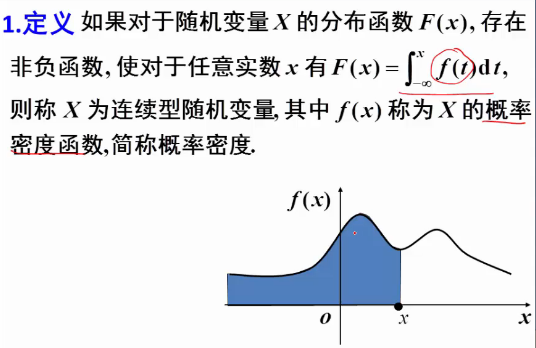2.性质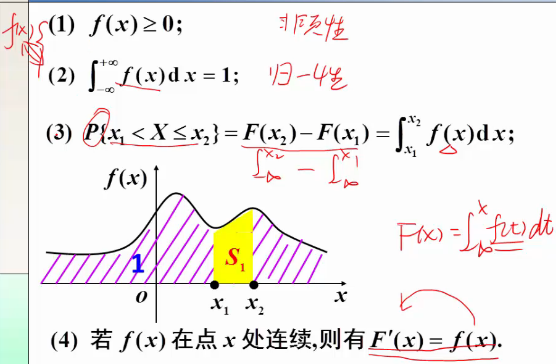典型例题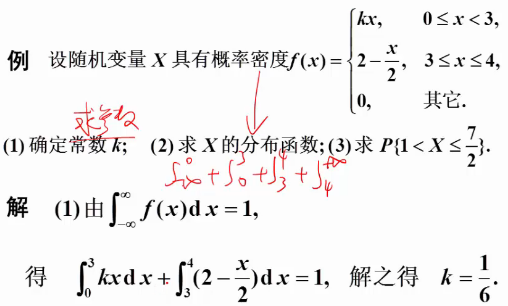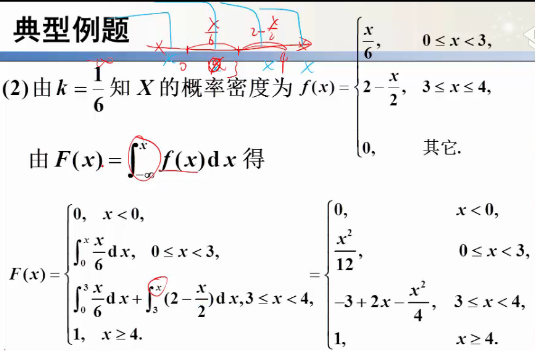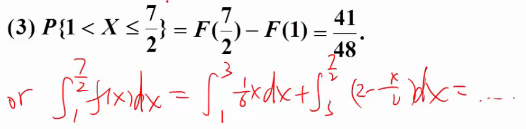展开全文• 总结的比较全的对与常见连续型分布函数，概率密度函数和特征函数的性质，并举出一些例题，是一个很好的参考资料
• 因为关于二维随机变量主题内容重要，难度大，例题多，最主要是积分区间...求二维函数Z=g(X,Y)型，用卷积公式求概率密度，积分区域如何确定（中） #### ======= 【例二】 设二维随机变量(X,Y)的概率密度为f(x...
因为关于二维随机变量主题内容重要，难度大，例题多，最主要是积分区间的确定是难点，同时关联卷积概念，卷积公式容易，积分区间难以确定，因为书上的例题都没有详细解释积分区间如何确定，所以分成上中下三篇博客写。
接本主题（上）。

求二维函数Z=g(X,Y)型，用卷积公式求概率密度，积分区域如何确定（中）

 ####  =======


【例二】

设二维随机变量(X,Y)的概率密度为f(x,y)={ 2−x−y,0<x<1,0<y<1, 0,othersf(x,y)= \begin{cases}
& \text{ } 2-x-y,   0< x< 1, 0< y< 1, \\
& \text{ } 0,    others
\end{cases}
(1)求P{X>2Y}
(2)求Z=X+Y的密度函数fz(z)${f}_{z}\left(z\right)$$f_{z}(z)$

【解】

(1)求P{X>2Y}

一般的解法：P(f(X,Y))总是通过概率分布FZ(z)${F}_{Z}\left(z\right)$$F_{Z}(z)$得来的，而 FZ(z)${F}_{Z}\left(z\right)$$F_{Z}(z)$又是通过概率密度积分得出来的。概率密度 fZ(z)=∫∞−∞f(x,z−x)dx${f}_{Z}\left(z\right)={\int }_{-\mathrm{\infty }}^{\mathrm{\infty }}f\left(x,z-x\right)dx$$f_{Z}(z) = \int_{-\infty}^{\infty}f(x,z-x)dx$
要求P{X>2Y}，
设Z=X-2Y, 求P(X>2Y)就是求P(Z>0)=1−FZ(z⩽0)$P\left(Z>0\right)=1-{F}_{Z}\left(z⩽0\right)$$P(Z>0) = 1-F_{Z}(z\leqslant 0)$
先求概率密度 fZ(z)=∫∞−∞f(x,z−x)dx${f}_{Z}\left(z\right)={\int }_{-\mathrm{\infty }}^{\mathrm{\infty }}f\left(x,z-x\right)dx$$f_{Z}(z) = \int_{-\infty}^{\infty}f(x,z-x)dx$

如上所述，如果按照先求概率密度的方法来算一个具体的特定的概率，求概率密度再求分布函数，会比较麻烦，对于一个特定的概率，可以通过画图图解法，看看积分区域在哪里，直接求dxdy的双重积分，也许对于特定概率来说更加方便。

对本题P(X>2Y) ，直接画一个x,y的坐标图，很容易看出来X>2Y的区域在哪里，再结合题目规定的区间  0< x< 1, 0< y< 1, 很容易算出，dxdy的双重积分。
在x,y的坐标系上，直接画一条Y=12X$Y=\frac{1}{2}X$$Y=\frac{1}{2}X$的直线，题目要求X>2Y，那么就是Y<\frac{1}{2}X，很容易看出来区域在哪里。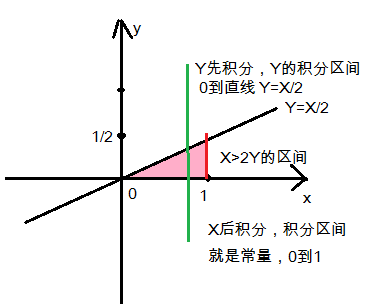图中粉红色的三角形区域就是X>2Y的区间，其中x限制在0到1，y也限制在0到1
先对Y积分，Y的积分区间就是0到直线Y=X/2；后对X积分，X的积分区间就是常量：0到1
P(X>2Y)=∫∫X>2Yf(x,y)dxdy$P\left(X>2Y\right)=\int {\int }_{X>2Y}f\left(x,y\right)dxdy$$P(X>2Y)=\int \int _{X>2Y} f(x,y)dxdy$
=∫10dx∫x20f(x,y)dy$={\int }_{0}^{1}dx{\int }_{0}^{\frac{x}{2}}f\left(x,y\right)dy$$=\int_{0}^{1}dx\int_{0}^{\frac{x}{2}}f(x,y)dy$
=∫10dx∫x20(2−x−y)dy=∫10dx((2−x)y−y22)|2x0$={\int }_{0}^{1}dx{\int }_{0}^{\frac{x}{2}}\left(2-x-y\right)dy={\int }_{0}^{1}dx\left(\left(2-x\right)y-\frac{{y}^{2}}{2}\right){|}_{0}^{\frac{2}{x}}$$=\int_{0}^{1}dx\int_{0}^{\frac{x}{2}}(2-x-y)dy = \int_{0}^{1}dx ((2-x)y-\frac{y^2}{2})|^\frac{2}{x}_0$
=∫10(x−58x2)dx=(x22−58∗x33)|10=12−58∗13=724$={\int }_{0}^{1}\left(x-\frac{5}{8}{x}^{2}\right)dx=\left(\frac{{x}^{2}}{2}-\frac{5}{8}\ast \frac{{x}^{3}}{3}\right){|}_{0}^{1}=\frac{1}{2}-\frac{5}{8}\ast \frac{1}{3}=\frac{7}{24}$$=\int_{0}^{1}(x-\frac{5}{8}x^2)dx = (\frac{x^2}{2}-\frac{5}{8}*\frac{x^3}{3})|^1_0=\frac{1}{2}-\frac{5}{8}*\frac{1}{3}=\frac{7}{24}$

(2)求Z=X+Y的密度函数fz(z)${f}_{z}\left(z\right)$$f_{z}(z)$

画出x-z坐标，算出两条直线范围，
Zmin=X+Ymin =X+0
Zmax=X+Ymax =X+1
加上X的0到1区间范围，就构成积分区间。如图，积分区间就是红色三角块和蓝色三角块。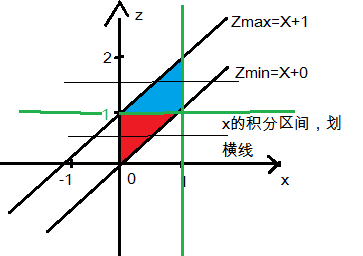fZ(z)=∫∞−∞f(x,z−x)dx${f}_{Z}\left(z\right)={\int }_{-\mathrm{\infty }}^{\mathrm{\infty }}f\left(x,z-x\right)dx$$f_{Z}(z) = \int_{-\infty}^{\infty}f(x,z-x)dx$
因此，积分区间按照z轴从下到上分，

当z<0,  由于z不在Zmin到Zmax的范围内，所以 fZ(z)=0${f}_{Z}\left(z\right)=0$$f_{Z}(z)=0$

当0⩽z<1$0⩽z<1$$0\leqslant z<1$,  对x先积分，x的积分区间为红色区块，x的边界为0到Zmin直线，所以积分区间就是0到z
fZ(z)=∫z0(2−x−(z−x))dx=∫z0(2−z)dx=(2−z)x|z0=(2−z)∗z${f}_{Z}\left(z\right)={\int }_{0}^{z}\left(2-x-\left(z-x\right)\right)dx={\int }_{0}^{z}\left(2-z\right)dx=\left(2-z\right)x{|}_{0}^{z}=\left(2-z\right)\ast z$$f_{Z}(z)=\int_{0}^{z}(2-x-(z-x))dx=\int_{0}^{z}(2-z)dx=(2-z)x|^z_0=(2-z)*z$

当1⩽z<2$1⩽z<2$$1\leqslant z<2$,  对x先积分，x的积分区间为蓝色区块，x的边界为Zmax直线到1，所以积分区间就是z-1到1
fZ(z)=∫1z−1(2−x−(z−x))dx=∫1z−1(2−z)dx=(2−z)x|1z−1=(2−z)2${f}_{Z}\left(z\right)={\int }_{z-1}^{1}\left(2-x-\left(z-x\right)\right)dx={\int }_{z-1}^{1}\left(2-z\right)dx=\left(2-z\right)x{|}_{z-1}^{1}=\left(2-z{\right)}^{2}$$f_{Z}(z)=\int_{z-1}^{1}(2-x-(z-x))dx=\int_{z-1}^{1}(2-z)dx=(2-z)x|^1_{z-1}=(2-z)^2$

所以，概率密度fZ(z)=⎧⎩⎨ z(2−z),0⩽z<1 (2−z)2,1⩽z<2 0,elsef_{Z}(z) =
\begin{cases}
& \text{ } z(2-z),0\leqslant z<1 \\
& \text{ } (2-z)^2,1\leqslant z<2 \\
& \text{ } 0, else
\end{cases}

####  =====


【例三】

假设一电路装有3个同种电气元件，其工作状态相互独立，且无故障工作时间都服从参数为λ>0$\lambda >0$$\lambda > 0$的指数分布，当3个元件都无故障时，电路正常工作，否则整个电路不能正常工作，试求电路正常工作的时间T的概率分布。

【解】
分布函数的物理含义：F(x)表示在x范围内（X⩽x)发生事件的概率。$F\left(x\right)表示在x范围内（X⩽x\right)发生事件的概率。$${\color{Red}{F(x)表示在x范围内（X\leqslant x)发生事件的概率。}}$

指数分布函数的含义：F(t)=1−e−λt，F(t)表示x时间前发生故障的概率。$F\left(t\right)=1-{e}^{-\lambda t}，F\left(t\right)表示x时间前发生故障的概率。$${\color{Red}{F(t)=1-e^{-\lambda t}，F(t)表示x时间前发生故障的概率。}}$

指数(概率密度)函数的意义：f(t)=e−λt，表示t时间内不发生故障的概率。$f\left(t\right)={e}^{-\lambda t}，表示t时间内不发生故障的概率。$${\color{Red}{f(t)=e^{-\lambda t}，表示t时间内不发生故障的概率。}}$

[分析]
关于指数分布，回顾一下博客离散型随机变量，二项分布，泊松分布，指数分布，几何分布（概统2.知识）
泊松分布是求某个时间t内，事件发生k次的概率
P{ X=k }=(λt)kk!∗e−λt$\frac{\left(\lambda t{\right)}^{k}}{k!}\ast {e}^{-\lambda t}$$\frac{ ({\lambda t})^k} {k!}*e^{-\lambda t}$
“所谓指数分布就是求事件发生的时间间隔”
P(X=0,N(t))=(λt)00!∗e−λt=e−λt$P\left(X=0,N\left(t\right)\right)=\frac{\left(\lambda t{\right)}^{0}}{0!}\ast {e}^{-\lambda t}={e}^{-\lambda t}$$P(X=0,N(t)) =\frac{(\lambda t)^0}{0!}*e^{-\lambda t}=e^{-\lambda t}$
“在t时间内出现一个以上的概率”
P{X>0,N(t)}=1−e−λt$P\left\{X>0,N\left(t\right)\right\}=1-{e}^{-\lambda t}$$P\{ X>0,N(t) \}=1-e^{-\lambda t}$

指数分布，
概率密度函数为f(x)=λe−λx$f\left(x\right)=\lambda {e}^{-\lambda x}$$f(x)=\lambda e^{-\lambda x}$
分布函数为F(x)=1−e−λx$F\left(x\right)=1-{e}^{-\lambda x}$$F(x)=1-e^{-\lambda x}$
简写 X∼E(λ)$X\sim E\left(\lambda \right)$$X \sim E(\lambda)$
参数λ$\lambda$$\lambda$为单位时间内事件发生的次数，即（电子元器件损坏的次数）。
所以电子元器件的平均寿命就是 指数分布的期望值 EX=1λ$EX=\frac{1}{\lambda }$$EX=\frac{1}{\lambda}$
假设某电子元器件的平均寿命为1000小时，那么EX=1000,λ=0.001$EX=1000,\lambda =0.001$$EX=1000, \lambda=0.001$,
某个电子元器件在1000小时内损坏的概率就是分布函数F(x<1000)
F(x<1000)=1−e−λx=1−1eλx$F\left(x<1000\right)=1-{e}^{-\lambda x}=1-\frac{1}{{e}^{\lambda x}}$$F(x<1000)=1-e^{-\lambda x} =1-\frac{1}{e^{\lambda x}}$
=1−1e0.001∗1000=1−1e$=1-\frac{1}{{e}^{0.001\ast 1000}}=1-\frac{1}{e}$$= 1 - \frac{1}{e^{0.001*1000}} = 1-\frac{1}{e}$

分布函数的物理含义：F(x)表示在x范围内（X⩽x)发生事件的概率。$F\left(x\right)表示在x范围内（X⩽x\right)发生事件的概率。$${\color{Red}{F(x)表示在x范围内（X\leqslant x)发生事件的概率。}}$

指数分布函数的含义：F(t)=1−e−λt$F\left(t\right)=1-{e}^{-\lambda t}$$F(t)=1-e^{-\lambda t}$，F(t)表示x时间前发生故障的概率。$F\left(t\right)表示x时间前发生故障的概率。$${\color{Red}{F(t)表示x时间前发生故障的概率。}}$

指数(概率密度)函数的意义：f(t)=e−λt$f\left(t\right)={e}^{-\lambda t}$$f(t)=e^{-\lambda t}$，表示t时间内不发生故障的概率。$表示t时间内不发生故障的概率。$${\color{Red}{表示t时间内不发生故障的概率。}}$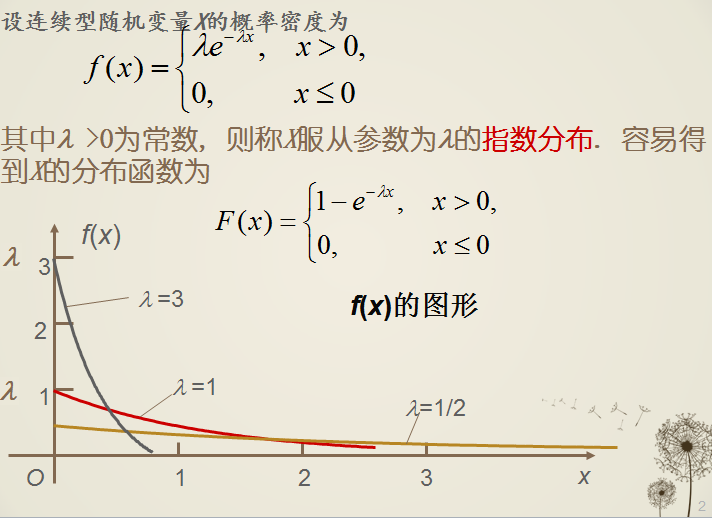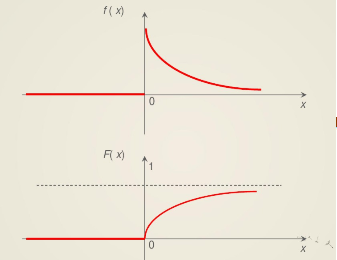=====
回到本题，
指数分布的分布函数是
分布函数为F(x)=1−e−λx$F\left(x\right)=1-{e}^{-\lambda x}$$F(x)=1-e^{-\lambda x}$
其意义是指在x时间内事件发生的概率，其等于t时间内出现一个以上的概率，等于P{X>0,N(t)}=1−e−λt$其意义是指在x时间内事件发生的概率，其等于t时间内出现一个以上的概率，等于P\left\{X>0,N\left(t\right)\right\}=1-{e}^{-\lambda t}$${\color{Red} {其意义是指在 x时间内事件发生的概率}}，其等于t 时间内出现一个以上的概率，等于P\{ X>0,N(t) \}=1-e^{-\lambda t}$。

本题求系统正常工作的时间的概率分布，
已知一个元件正常工作的概率分布（t时间内发生故障的概率）F(t)=1−e−λt$F\left(t\right)=1-{e}^{-\lambda t}$$F(t)=1-e^{-\lambda t}$
系统正常工作，要求三个元件都不发生故障，F(t)是发生故障的概率，不发生故障的概率是1-F(t), 如果是三个F(t)相乘，等于三个同时发生故障的概率，一个都不发生故障，应该是三个(1-F(t))相乘，系统要求求三个都不发生故障的分布函数，就是1-三个（1-F(t))相乘。

所以
FT(t)=1−（1−F1(t)）∗（1−F2(t)）∗（1−F3(t)）${F}_{T}\left(t\right)=1-（1-{F}_{1}\left(t\right)）\ast （1-{F}_{2}\left(t\right)）\ast （1-{F}_{3}\left(t\right)）$$F_{T}(t) = 1-（1-F_{1}(t)）*（1-F_{2}(t)）*（1-F_{3}(t)）$
=1−(e−λt)3$=1-\left({e}^{-\lambda t}{\right)}^{3}$$=1 - ( e^{-\lambda t}) ^3$              (1)

如果是三个F_{T}(t)直接相乘，得到的结果是
FT1(t)=F1(t)∗F2(t)∗F3(t)${F}_{T1}\left(t\right)={F}_{1}\left(t\right)\ast {F}_{2}\left(t\right)\ast {F}_{3}\left(t\right)$$F_{T1}(t) = F_{1}(t)*F_{2}(t)*F_{3}(t)$
=(1−e−λt)3$=\left(1-{e}^{-\lambda t}{\right)}^{3}$$= ( 1-e^{-\lambda t}) ^3$
=1−3e−λt+3e−2λt−e3λt$1-3{e}^{-\lambda t}+3{e}^{-2\lambda t}-{e}^{3\lambda t}$$1-3e^{-\lambda t}+3e^{-2\lambda t} -e^{3\lambda t}$ = 1−3e−λt(1−e−λt)−e3λt$1-3{e}^{-\lambda t}\left(1-{e}^{-\lambda t}\right)-{e}^{3\lambda t}$$1-3e^{-\lambda t}(1-e^{-\lambda t})-e^{3\lambda t}$    (2)
其中，(1−e−λt)$\left(1-{e}^{-\lambda t}\right)$$(1-e^{-\lambda t})$ 肯定是大于0 的，所以(2)式的整体值小于(1)式，
FT1(t)${F}_{T1}\left(t\right)$$F_{T1}(t)$ 小于 FT(t)${F}_{T}\left(t\right)$$F_{T}(t)$ ， 而F(t)的含义是在t时间前系统发生故障的概率， 而实际上要求三个元件同时不发生故障系统才算不发生故障， 所以F(t)应该取大才对。

还有一个公式
在书上P82(2).3${P}_{82}\left(2\right).3$$P_{82}(2).3$：
设X,Y相互独立，分布函数分别为F_{X}(x)和F_{Y}(y)，M=max(X.Y),  N=min(X,Y)，则
FM(z)=FX(z)∗FY(z),FN(z)=1−[1−FX(z)][1−FY(z)]${F}_{M}\left(z\right)={F}_{X}\left(z\right)\ast {F}_{Y}\left(z\right),{F}_{N}\left(z\right)=1-\left[1-{F}_{X}\left(z\right)\right]\left[1-{F}_{Y}\left(z\right)\right]$$F_{M}(z)=F_{X}(z)*F_{Y}(z) , F_{N}(z)=1-[1-F_{X}(z)][1-F_{Y}(z)]$

本题中 T=min(X1, X2, X3)，故 G(t)=1−[1−F(t)]3$G\left(t\right)=1-\left[1-F\left(t\right){\right]}^{3}$$G(t)=1-[1-F(t)]^3$

【例四】

设随机变量(X,Y)在矩形区域G=((x,y)|0⩽x⩽2,0⩽y⩽1)$G=\left(\left(x,y\right)|0⩽x⩽2,0⩽y⩽1\right)$$G=((x,y)|0\leqslant x\leqslant 2,0\leqslant y\leqslant 1)$上服从均匀分布，试求Z=XY的密度函数。

【 解 】

需要理解的是：二维随机变量均匀分布的概率密度函数=1分布区域的面积$二维随机变量均匀分布的概率密度函数=\frac{1}{分布区域的面积}$${\color{Red} {二维随机变量均匀分布的概率密度函数 = \frac{1}{分布区域的面积} }}$

区域G的面积=x的长度乘以y的宽度=(2-0) x (1-0)=2*1=2

X和Y的均匀分布的概率密度函数 = 1分布区域的面积=12$\frac{1}{分布区域的面积}=\frac{1}{2}$$\frac{1}{分布区域的面积}=\frac{1}{2}$

区域G的面积=x的长度y的宽度=(2-0)(1-0)
均匀分布的密度函数 = 1区域G的面积=12∗1=12$\frac{1}{区域G的面积}=\frac{1}{2\ast 1}=\frac{1}{2}$$\frac{1}{区域G的面积} = \frac{1}{2*1} = \frac{1}{2}$

对于 Z=X*Y的密度函数与Z=X+Y的密度函数有所不同。
见P82.(2)

Z=X+Y型的密度函数为，fZ(z)=∫∞−∞f(x,z−x)dx$Z=X+Y型的密度函数为，{f}_{Z}\left(z\right)={\int }_{-\mathrm{\infty }}^{\mathrm{\infty }}f\left(x,z-x\right)dx$${\color{Red} {Z=X+Y型的密度函数为，f_{Z}(z)=\int_{-\infty}^{\infty}f(x,z-x)dx}}$

Z=X/Y型的密度函数为，fY/X(z)=∫∞−∞|x|f(x,zx)dx$Z=X/Y型的密度函数为，{f}_{Y/X}\left(z\right)={\int }_{-\mathrm{\infty }}^{\mathrm{\infty }}|x|f\left(x,zx\right)dx$${\color{Red} {Z=X/Y型的密度函数为，f_{Y/X}(z)=\int_{-\infty}^{\infty}|x|f(x,zx)dx}}$

Z=XY型的密度函数为，fYX(z)=∫∞−∞1|x|f(x,zx)dx$Z=XY型的密度函数为，{f}_{YX}\left(z\right)={\int }_{-\mathrm{\infty }}^{\mathrm{\infty }}\frac{1}{|x|}f\left(x,\frac{z}{x}\right)dx$${\color{Red} {Z=XY型的密度函数为，f_{YX}(z)=\int_{-\infty}^{\infty}\frac{1}{|x|}f(x,\frac{z}{x})dx}}$

对于本题，要求Z=XY的密度函数
Z=XY型的密度函数为，fYX(z)=∫∞−∞1|x|f(x,zx)dx$Z=XY型的密度函数为，{f}_{YX}\left(z\right)={\int }_{-\mathrm{\infty }}^{\mathrm{\infty }}\frac{1}{|x|}f\left(x,\frac{z}{x}\right)dx$$Z=XY型的密度函数为，f_{YX}(z)=\int_{-\infty}^{\infty}\frac{1}{|x|}f(x,\frac{z}{x})dx$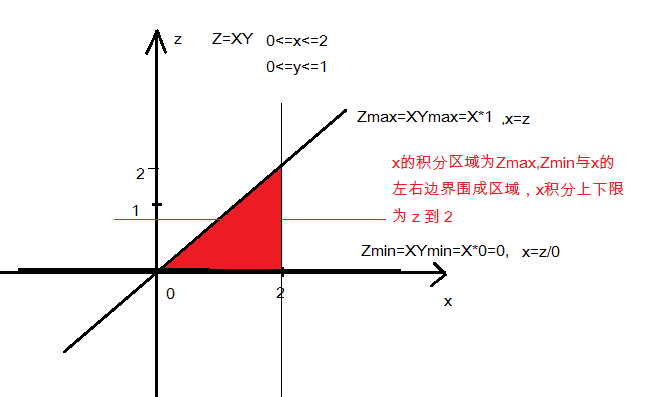如图所示，
Zmax = X*Ymax = X*1 =X, ==> 直线边界：x=z
Zmin = X*Ymin = X*0 =0 , ==> 直线边界：z=0
X的左右边界为 0 到 2
所以积分区域就是Zmax, Zmin和X的左右边界围成的区块

对x进行积分，x的左边界为Zmax直线，即x=z，右边界为x=2垂直直线。
当 0⩽z<2时，fXY(z)=∫∞−∞1|x|f(x,zx)dx=∫2z1|x|∗12dx$0⩽z<2时，{f}_{XY}\left(z\right)={\int }_{-\mathrm{\infty }}^{\mathrm{\infty }}\frac{1}{|x|}f\left(x,\frac{z}{x}\right)dx={\int }_{z}^{2}\frac{1}{|x|}\ast \frac{1}{2}dx$$0\leqslant z<2 时， f_{XY}(z)=\int_{-\infty}^{\infty}\frac{1}{|x|}f(x,\frac{z}{x})dx = \int_{z}^{2}\frac{1}{|x|}*\frac{1}{2}dx$
博客积分公式小节
基本积分表中有：∫dxx=ln|x|+C$\int \frac{dx}{x}=ln|x|+C$$\int \frac{dx}{x}=ln|x|+C$
得，
fXY(z)={12(ln2−lnz)0,0⩽z<2,else${f}_{XY}\left(z\right)=\left\{\begin{array}{ccc}& \frac{1}{2}\left(ln2-lnz\right)& ,0⩽z<2\\ & 0& ,else\end{array}$f_{XY}(z)= \left\{\begin{matrix}
&\frac{1}{2}(ln2-lnz) & ,0\leqslant z<2\\
&0  &,else
\end{matrix}\right.

参考书目：

张天德，叶宏 《星火燎原·概率论与数理统计辅导及习题精解》（浙大·第4版）第三章
展开全文卷积公式
• 因为关于二维随机变量主题内容重要，难度大，例题多，最主要是积分区间的确定是难点，同时关联卷积概念，求二维函数Z=g(X,Y)型，用卷积公式求概率密度，卷积公式容易，积分区间难以确定，所以分成上中下三篇博客写。...
二维函数Z=g(X,Y)型，用卷积公式求概率密度，积分区域如何确定（上）

因为关于二维随机变量主题内容重要，难度大，例题多，最主要是积分区间的确定是难点，同时关联卷积概念，求二维函数Z=g(X,Y)型，用卷积公式求概率密度，卷积公式容易，积分区间难以确定，所以分成上中下三篇博客写。

一。问题的引入

有一大群人，令X和Y分别表示一个人的年龄和体重，Z表示该人的血压，并且已知Z与X,Y的关系为 Z=g(X,Y), 如何通过X,Y的分布确定Z的分布？二。公式

Fz(z)=P(Z⩽z)=∫∫g(x,y)⩽zf(x,y)dxdy${F}_{z}\left(z\right)=P\left(Z⩽z\right)=\int {\int }_{g\left(x,y\right)⩽z}f\left(x,y\right)dxdy$$F_{z}(z)=P(Z\leqslant z)=\int \int_{g(x,y)\leqslant z} f(x,y)dxdy$

特殊类型：Z=X+Y，怎样确定Z的分布？如何求Z的概率密度？
fz(z)=∫∞−∞f(x,z−x)dx=∫∞−∞f(z−y,y)dy${f}_{z}\left(z\right)={\int }_{-\mathrm{\infty }}^{\mathrm{\infty }}f\left(x,z-x\right)dx={\int }_{-\mathrm{\infty }}^{\mathrm{\infty }}f\left(z-y,y\right)dy$$f_{z}(z)=\int_{-\infty }^{\infty }f(x,z-x)dx = \int_{-\infty }^{\infty }f(z-y,y)dy$

当X与Y相互独立时，
就得到所谓的卷积公式$卷积公式$${\color{Red} {卷积公式}}$
fz(z)=fx∗fy=∫∞−∞fX(x)fY(z−x)dx=∫∞−∞fX(z−y)fY(y)dy${f}_{z}\left(z\right)={f}_{x}\ast {f}_{y}={\int }_{-\mathrm{\infty }}^{\mathrm{\infty }}{f}_{X}\left(x\right){f}_{Y}\left(z-x\right)dx={\int }_{-\mathrm{\infty }}^{\mathrm{\infty }}{f}_{X}\left(z-y\right){f}_{Y}\left(y\right)dy$$f_{z}(z)=f_{x}*f_{y}=\int_{-\infty }^{\infty }f_{X}(x)f_{Y}(z-x)dx = \int_{-\infty }^{\infty }f_{X}(z-y)f_{Y}(y)dy$
这就是所谓的卷积积分

三。已知f(x,y)，如何计算Z=X+Y型的概率密度fz(z)${f}_{z}\left(z\right)$$f_{z}(z)$ 及概率分布 Fz(z)${F}_{z}\left(z\right)$$F_{z}(z)$？

根据理解或者根据上面的公式，我们知道 fz(z)${f}_{z}\left(z\right)$$f_{z}(z)$ 是将f(x,y)求一次积分，Fz(z)${F}_{z}\left(z\right)$$F_{z}(z)$是求二次积分，难点问题在于如何确定积分区间？需要分成几个区间？$如何确定积分区间？需要分成几个区间？$${\color{Red} {如何确定积分区间？需要分成几个区间？}}$

对于Z=X+Y型的关系，假设对x求一次积分，得到fz(z)${f}_{z}\left(z\right)$$f_{z}(z)$
表示成

fz(z)=∫∞−∞f(x,z−x)dx${f}_{z}\left(z\right)={\int }_{-\mathrm{\infty }}^{\mathrm{\infty }}f\left(x,z-x\right)dx$$f_{z}(z)=\int_{-\infty }^{\infty }f(x,z-x)dx$

，那么我们要画出一个 x–z的坐标，确定积分区间

1）积分区间的左右两边，由x的上下区间决定
假设 x的区间在[a,b]之间, a⩽x⩽b$a⩽x⩽b$$a\leqslant x\leqslant b$
那么积分的左右边界就是a到b
2）根据关系式
z=x+y， 由于坐标系是x–z的关系，那么y就是变常量
z的最小值：zmin=x+ymin${z}_{min}=x+{y}_{min}$$z_{min}=x+y_{min}$
z的最大值：zmax=x+ymax${z}_{max}=x+{y}_{max}$$z_{max}=x+y_{max}$
积分的上下边界就是 zmin${z}_{min}$$z_{min}$到 zmax${z}_{max}$$z_{max}$

因为我们讨论的f_{z}(z)是按照x积分：
fz(z)=∫∞−∞f(x,z−x)dx${f}_{z}\left(z\right)={\int }_{-\mathrm{\infty }}^{\mathrm{\infty }}f\left(x,z-x\right)dx$$f_{z}(z)=\int_{-\infty }^{\infty }f(x,z-x)dx$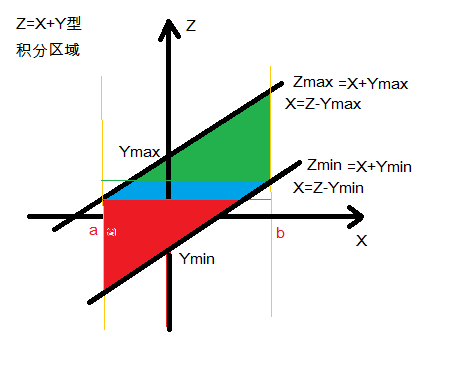所以按照x积分，积分区间就要分成三段：红色区间，蓝色区间，绿色区间

1)红色区间$红色区间$${\color{Red} {红色区间}}$，
Xmin+Ymin⩽z<Xmin+Ymax${X}_{min}+{Y}_{min}⩽z<{X}_{min}+{Y}_{max}$$X_{min}+Y_{min} \leqslant z
x积分区间= a 到 Z-Ymin
∫z−yminadx${\int }_{a}^{z-{y}_{min}}dx$$\int_{a}^{z-y_{min}}dx$

2)蓝色区间$蓝色区间$${\color{Blue} {蓝色区间}}$，
Xmin+Ymax⩽z<Xmax+Ymin${X}_{min}+{Y}_{max}⩽z<{X}_{max}+{Y}_{min}$$X_{min}+Y_{max} \leqslant z
x积分区间= Z-Ymax 到 Z-Ymin
∫z−yminz−ymaxdx${\int }_{z-{y}_{max}}^{z-{y}_{min}}dx$$\int_{z-y_{max}}^{z-y_{min}}dx$

3)绿色区间$绿色区间$${\color{Green} {绿色区间}}$，
Xmax+Ymin⩽z<Xmax+Ymax${X}_{max}+{Y}_{min}⩽z<{X}_{max}+{Y}_{max}$$X_{max}+Y_{min} \leqslant z

x积分区间= Z-Ymax 到  1
∫bz−ymaxdx${\int }_{z-{y}_{max}}^{b}dx$$\int_{z-y_{max}}^{b}dx$

当x的a,b左右对称时，中间蓝色区间没有，只有两个积分区间：
红色区间$红色区间$${\color{Red} {红色区间}}$和 绿色区间$绿色区间$${\color{Green} {绿色区间}}$

=========================
【例一】设(X,Y)的联合密度函数为
f(x,y)={ e−y,0⩽x⩽1,y⩾0, 0,othersf(x,y)= \begin{cases}
& \text{ } e^{-y}, 0\leqslant x\leqslant 1, y\geqslant 0, \\
& \text{ } 0,    others
\end{cases}
(1)问X,Y是否独立？
(2)求Z=2X+Y的密度函数fz(z)${f}_{z}\left(z\right)$$f_{z}(z)$和分布函数Fz(z)${F}_{z}\left(z\right)$$F_{z}(z)$
(3)求P{Z>3}

【解】
(1) 问X,Y是否独立？
X,Y独立的条件 f(x,y)=fx(x)∗fy(y)$f\left(x,y\right)={f}_{x}\left(x\right)\ast {f}_{y}\left(y\right)$$f(x,y)=f_{x}(x)*f_{y}(y)$
fX(x)=∫∞−∞f(x,y)dy${f}_{X}\left(x\right)={\int }_{-\mathrm{\infty }}^{\mathrm{\infty }}f\left(x,y\right)dy$$f_{X}(x)=\int_{-\infty }^{\infty}f(x,y)dy$
fX(x)=∫∞0e−ydy=−e−y|∞0=e−y|0∞=1${f}_{X}\left(x\right)={\int }_{0}^{\mathrm{\infty }}{e}^{-y}dy=-{e}^{-y}{|}_{0}^{\mathrm{\infty }}={e}^{-y}{|}_{\mathrm{\infty }}^{0}=1$$f_{X}(x)=\int_{0 }^{\infty}e^{-y}dy=-e^{-y}|^\infty_{0}=e^{-y}|^0_{\infty}=1$

fY(y)=∫∞−∞f(x,y)dx${f}_{Y}\left(y\right)={\int }_{-\mathrm{\infty }}^{\mathrm{\infty }}f\left(x,y\right)dx$$f_{Y}(y)=\int_{-\infty }^{\infty}f(x,y)dx$
fY(y)=∫∞0e−ydx=∫10e−ydx=e−y∗(x)|10=e−y${f}_{Y}\left(y\right)={\int }_{0}^{\mathrm{\infty }}{e}^{-y}dx={\int }_{0}^{1}{e}^{-y}dx={e}^{-y}\ast \left(x\right){|}_{0}^{1}={e}^{-y}$$f_{Y}(y)=\int_{0 }^{\infty}e^{-y}dx= \int_{0 }^{1}e^{-y}dx=e^{-y}*(x)|^1_{0}=e^{-y}$
所以 f(x,y)=fX(x)∗fY(y)$f\left(x,y\right)={f}_{X}\left(x\right)\ast {f}_{Y}\left(y\right)$$f(x,y)=f_{X}(x)*f_{Y}(y)$

(2)求Z=2X+Y的密度函数fz(z)${f}_{z}\left(z\right)$$f_{z}(z)$和分布函数Fz(z)${F}_{z}\left(z\right)$$F_{z}(z)$

(2.1)先求密度函数fz(z)${f}_{z}\left(z\right)$$f_{z}(z)$

Z=g(X,Y)=2X+Y

求fz(z)${f}_{z}\left(z\right)$$f_{z}(z)$可以利用卷积公式
fZ(z)=∫∞−∞f(x,z−x)dx${f}_{Z}\left(z\right)={\int }_{-\mathrm{\infty }}^{\mathrm{\infty }}f\left(x,z-x\right)dx$$f_{Z}(z)=\int_{-\infty}^{\infty}f(x,z-x)dx$

画一个 x-z 的坐标系

Z方向下限：
Zmin=g(X,Ymin)=2X+Ymin${Z}_{min}=g\left(X,{Y}_{min}\right)=2X+{Y}_{min}$$Z_{min}=g(X,Y_{min})=2X+Y_{min}$ = 2X+0

Z方向上限：
Zmin=g(X,Ymin)=2X+Ymax=2X+∞=∞${Z}_{min}=g\left(X,{Y}_{min}\right)=2X+{Y}_{max}=2X+\mathrm{\infty }=\mathrm{\infty }$$Z_{min}=g(X,Y_{min})=2X+Y_{max} = 2X+\infty =\infty$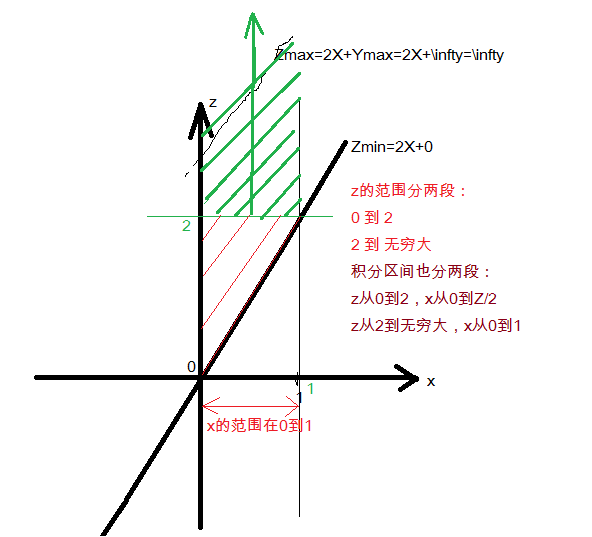所以，对公式 fZ(z)=∫∞−∞f(x,z−x)dx${f}_{Z}\left(z\right)={\int }_{-\mathrm{\infty }}^{\mathrm{\infty }}f\left(x,z-x\right)dx$$f_{Z}(z)=\int_{-\infty}^{\infty}f(x,z-x)dx$

当 0⩽z<2:0⩽x<z2$0⩽z<2:0⩽x<\frac{z}{2}$$0\leqslant z < 2 : 0\leqslant x < \frac{z}{2}$
fZ(z)=∫z20f(x,z−x)dx=∫z20e−(z−2x)dx${f}_{Z}\left(z\right)={\int }_{0}^{\frac{z}{2}}f\left(x,z-x\right)dx={\int }_{0}^{\frac{z}{2}}{e}^{-\left(z-2x\right)}dx$$f_{Z}(z)=\int_{0}^{\frac{z}{2}}f(x,z-x)dx = \int_{0}^{\frac{z}{2}} e^{-(z-2x)}dx$
∫z20e2x−zdx(设t=2x−z,dt=2dx)${\int }_{0}^{\frac{z}{2}}{e}^{2x-z}dx\left(设t=2x-z,dt=2dx\right)$$\int_{0}^{\frac{z}{2}}e^{2x-z}dx (设t=2x-z, dt=2dx)$
=12e2x−z|z20$\frac{1}{2}{e}^{2x-z}{|}_{0}^{\frac{z}{2}}$$\frac{1}{2} e^{2x-z}|_0^\frac{z}{2}$
=12(1−e−z)$\frac{1}{2}\left(1-{e}^{-z}\right)$$\frac{1}{2}(1-e^{-z})$

当 2⩽z<∞:0⩽x⩽1$2⩽z<\mathrm{\infty }:0⩽x⩽1$$2\leqslant z < \infty : 0\leqslant x \leqslant 1$
fZ(z)=∫10f(x,z−x)dx=∫10e−(z−2x)dx${f}_{Z}\left(z\right)={\int }_{0}^{1}f\left(x,z-x\right)dx={\int }_{0}^{1}{e}^{-\left(z-2x\right)}dx$$f_{Z}(z)=\int_{0}^{1}f(x,z-x)dx = \int_{0}^{1} e^{-(z-2x)}dx$
∫10e2x−zdx(设t=2x−z,dt=2dx)${\int }_{0}^{1}{e}^{2x-z}dx\left(设t=2x-z,dt=2dx\right)$$\int_{0}^{1}e^{2x-z}dx (设t=2x-z, dt=2dx)$
=12e2x−z|10$\frac{1}{2}{e}^{2x-z}{|}_{0}^{1}$$\frac{1}{2} e^{2x-z}|_0^1$
=12(e2−1)e−z$\frac{1}{2}\left({e}^{2}-1\right){e}^{-z}$$\frac{1}{2}(e^2-1)e^{-z}$

所以 fZ(z)=⎧⎩⎨⎪⎪ 0,z<0, 12(1−e−z),0⩽z⩽2, 12(e2−1)e−z,z>2f_{Z}(z)=
\begin{cases}
& \text{ } 0,z<0, \\
& \text{ } \frac{1}{2}(1-e^{-z}), 0\leqslant z\leqslant 2, \\
& \text{ } \frac{1}{2}(e^2-1)e^{-z}, z> 2
\end{cases}

(2.2) 求分布函数Fz(z)${F}_{z}\left(z\right)$$F_{z}(z)$

由分布函数Fz(z)${F}_{z}\left(z\right)$$F_{z}(z)$的定义可以知道，就是对z再积分
Fz(z)${F}_{z}\left(z\right)$$F_{z}(z)$与相应的概率密度函数fZ(z)${f}_{Z}\left(z\right)$$f_{Z}(z)$的积分区间的关系是怎样呢？
概率密度函数fZ(z)${f}_{Z}\left(z\right)$$f_{Z}(z)$是对横坐标x积分，分布函数Fz(z)${F}_{z}\left(z\right)$$F_{z}(z)$是对纵坐标z进行积分。通过z进行分区段，Fz(z)${F}_{z}\left(z\right)$$F_{z}(z)$与fz(z)${f}_{z}\left(z\right)$$f_{z}(z)$是一样的，但是Fz(z)${F}_{z}\left(z\right)$$F_{z}(z)$是把fz(z)${f}_{z}\left(z\right)$$f_{z}(z)$再次对z求积分，z的上下限的取值与z的分段不完全一样。

对z积分上下限取值原则：z的取值一直是从小到大方向，下限固定，上限活动，上限就是z$对z积分上下限取值原则：z的取值一直是从小到大方向，下限固定，上限活动，上限就是z$${\color{Red} {对z积分上下限取值原则：z的取值一直是从小到大方向，下限固定，上限活动，上限就是z}}$

FZ(z)=∫∞−∞fZ(z)dz${F}_{Z}\left(z\right)={\int }_{-\mathrm{\infty }}^{\mathrm{\infty }}{f}_{Z}\left(z\right)dz$$F_{Z}(z)=\int_{-\infty}^{\infty}f_{Z}(z)dz$
根据fZ(z)${f}_{Z}\left(z\right)$$f_{Z}(z)$的分段，分段再积分
所以，
z<0 时，F_{Z}(z)=0
当 0⩽z<2$0⩽z<2$$0\leqslant z < 2$ : z的积分区间：下限固定，下限是0，上限活动，上限是z$下限固定，下限是0，上限活动，上限是z$${\color{Red} {下限固定，下限是0，上限活动，上限是z}}$，所以就是 在0⩽z<z$0⩽z$0\leqslant z < z$
FZ(z)=∫z0fZ(z)dz=∫z012(1−e−z)dz${F}_{Z}\left(z\right)={\int }_{0}^{z}{f}_{Z}\left(z\right)dz={\int }_{0}^{z}\frac{1}{2}\left(1-{e}^{-z}\right)dz$$F_{Z}(z)=\int_{0}^{z}f_{Z}(z)dz = \int_{0}^{z} \frac{1}{2}(1-e^{-z})dz$
=12(z+e−z)|z0=12(z−1+e−z)$\frac{1}{2}\left(z+{e}^{-z}\right){|}_{0}^{z}=\frac{1}{2}\left(z-1+{e}^{-z}\right)$$\frac{1}{2}(z+e^{-z})|_{0}^{z}=\frac{1}{2}(z-1+e^{-z})$

当 2⩽z<∞$2⩽z<\mathrm{\infty }$$2\leqslant z < \infty$ :  注意分布函数与密度函数的区别，分布函数是对z的累加，要把前面的所有区间全部累加起来$要把前面的所有区间全部累加起来$${\color{Red} {要把前面的所有区间全部累加起来 }}$
当 2⩽z<∞$2⩽z<\mathrm{\infty }$$2\leqslant z < \infty$：z的积分区间为前面一段区间： 0到2，再加上当前区间，下限固定，下限就是2，上限活动，上限就是z$下限固定，下限就是2，上限活动，上限就是z$${\color{Red} {下限固定，下限就是2，上限活动，上限就是z}}$

FZ(z)=∫20fZ(z)dz+∫z2fZ(z)dz=${F}_{Z}\left(z\right)={\int }_{0}^{2}{f}_{Z}\left(z\right)dz+{\int }_{2}^{z}{f}_{Z}\left(z\right)dz=$$F_{Z}(z)=\int_{0}^{2}f_{Z}(z)dz + \int_{2}^{z}f_{Z}(z)dz=$
=∫2012(1−e−z)dz+∫z212(e2−1)e−zdz$={\int }_{0}^{2}\frac{1}{2}\left(1-{e}^{-z}\right)dz+{\int }_{2}^{z}\frac{1}{2}\left({e}^{2}-1\right){e}^{-z}dz$$={\color{Red} {\int_{0}^{2}\frac{1}{2}(1-e^{-z}) dz}}+ \int_{2}^{z}\frac{1}{2}(e^2-1)e^{-z}dz$
=1+12(1−e2)e−z$=1+\frac{1}{2}\left(1-{e}^{2}\right){e}^{-z}$$=1+\frac{1}{2}(1-e^2)e^{-z}$

所以FZ(z)=⎧⎩⎨⎪⎪ 0,z<0 12(z−1+e−z),0⩽z<2 1+12(1−e2)e−z,z⩾2F_{Z}(z)=
\begin{cases}
& \text{  } 0, z<0 \\
& \text{  } \frac{1}{2}(z-1+e^{-z}),0\leqslant z<2 \\
& \text{  } 1+\frac{1}{2}(1-e^2)e^{-z}, z\geqslant 2
\end{cases}

(3)求P{Z>3}

求P(f(Z))总是跟分布函数FZ(z)${F}_{Z}\left(z\right)$$F_{Z}(z)$联系在一起的。根据概率分布函数的定义 FZ(z)${F}_{Z}\left(z\right)$$F_{Z}(z)$指的是从−∞$-\mathrm{\infty }$$-\infty$到当前z的累加，运算值和查表值都只是 −∞$-\mathrm{\infty }$$-\infty$到某个当前z值得积分，即，积分的结果表示的是 P(Z⩽z)$P\left(Z⩽z\right)$$P(Z\leqslant z)$的值

所以
P(Z>3)=1−P(Z⩽3)=1−FZ(z)(z=3)$P\left(Z>3\right)=1-P\left(Z⩽3\right)=1-{F}_{Z}\left(z\right)\left(z=3\right)$$P(Z>3)=1-P(Z\leqslant 3)=1-F_{Z}(z)(z=3)$

根据上面的积分结果
FZ(3)=(1+12(1−e2)e−z)|z=3${F}_{Z}\left(3\right)=\left(1+\frac{1}{2}\left(1-{e}^{2}\right){e}^{-z}\right){|}_{z=3}$$F_{Z}(3) = ( 1+\frac{1}{2}(1-e^2)e^{-z})|_{z=3}$

P(Z>3)=1−(1+12(1−e2))e−3$P\left(Z>3\right)=1-\left(1+\frac{1}{2}\left(1-{e}^{2}\right)\right){e}^{-3}$$P(Z>3) =1-(1+\frac{1}{2}(1-e^2))e^{-3}$
=12(e2−1)e−3≈0.1591$=\frac{1}{2}\left({e}^{2}-1\right){e}^{-3}\approx 0.1591$$= \frac{1}{2}(e^2-1)e^{-3} \approx 0.1591$

参考书目：

张天德，叶宏 《星火燎原·概率论与数理统计辅导及习题精解》（浙大·第4版）第三章
展开全文卷积公式
• 你会在时间区段[t1,t2],中的忍一时可以相同的概率密度到达，你的朋友则会在[s1,s2]中的任意时刻到达，你们的火车会在A市停留w分钟。输入格式：第一行为数据组数T，接下来的T行每行包含5个整数，分别为t...
• 二维函数Z=g(X,Y)型，用卷积公式求概率密度，积分区域如何确定（下） 因为关于二维随机变量主题内容重要，难度大，例题多，最主要是积分区间的确定是难点，同时关联卷积概念，卷积公式容易，积分区间难以确定，因为...卷积公式 二维随机变量